### 长安沃尔沃 沃尔沃S80L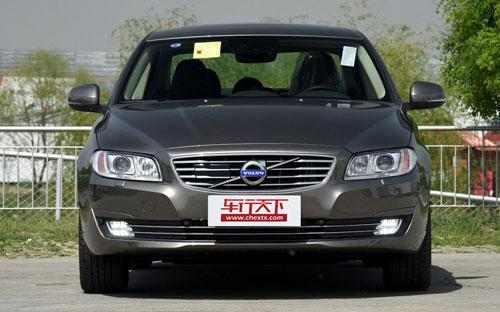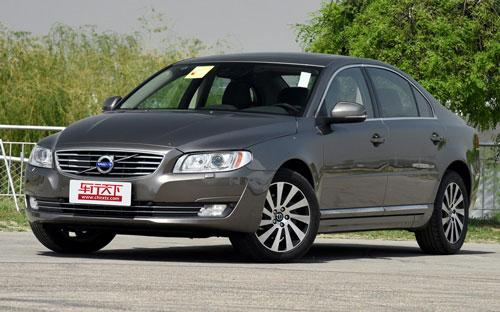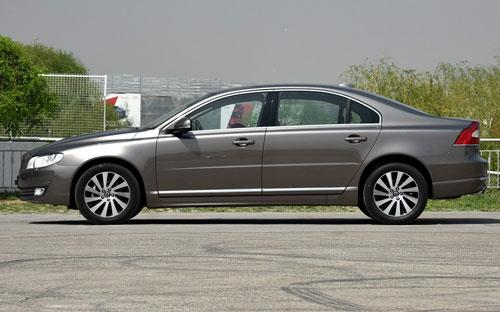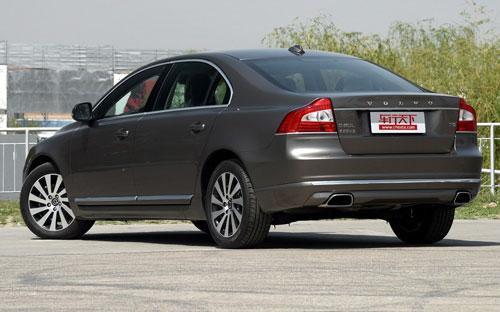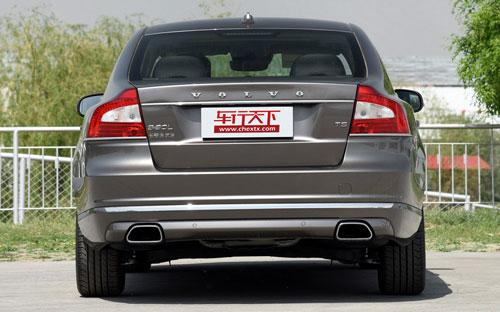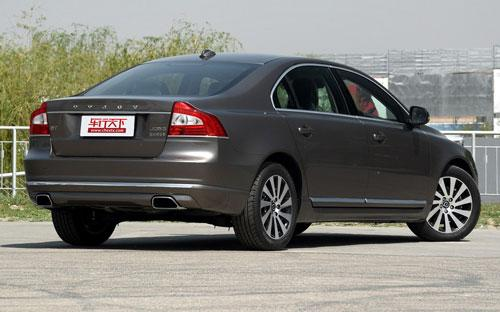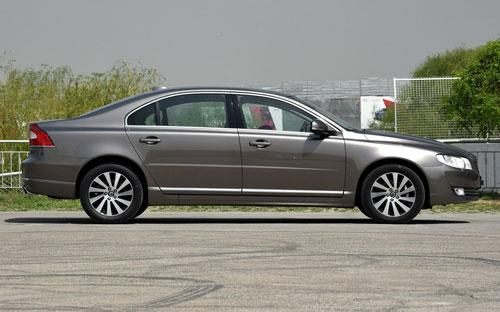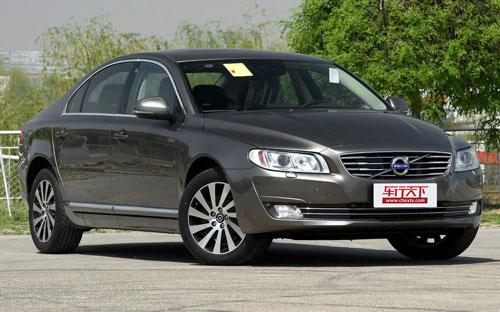4 种颜色可选2015款最低售价：39.99 万元起

4991(mm)1861(mm)1490(mm)##### 配置亮点：
• 胎压监测装置

• ISOFIX儿童座椅接口

• 车身稳定控制(ESC/ESP/DSC等)

• 电动天窗

• 定速巡航

• 后倒车雷达

• 真皮座椅

• GPS导航系统

• 氙气大灯

• 后视镜加热

• 提交
2015款 2.0T T5 智雅版 (146张)

长安沃尔沃 沃尔沃S80L 绕车实拍• 长安沃尔沃 沃尔沃S80L 在售车型

排量 车型 厂商指导价 本地最低报价 购车工具
2.0T
2.0T T5 智逸版 停产停售 6挡手自一体
39.99万
39.99万

2.0T T5 智雅版 停产停售 6挡手自一体
43.49万
43.49万

2.0T T5 智尊行政版 停产停售 6挡手自一体
49.99万
49.99万

长安沃尔沃 沃尔沃S80L 经销商

查看更多 >>

### 长安沃尔沃 沃尔沃S80L 动力加速

沃尔沃S80L 0-100公里加速时间分布在 8.1-8.1秒 属于 运动级

动力级别 加速时间 车型
运动级(1款)
8.1s
2.0T T5 智逸版2.0T T5 智雅版2.0T T5 智尊行政版

长安沃尔沃 沃尔沃S80L 视频

长安沃尔沃 沃尔沃S80L 新闻资讯

# 摆脱依赖 试驾评测新款沃尔沃S80L

评测 超过3758次关注

吉利接管沃尔沃已经三年了，尽管还没什么新车型，但至少这个独立的瑞典品牌正逐步摆脱对福特技术的依赖，如今包括S80L在内的主要车型都开始恢复采用经典的5缸发动机了。

# 成都车展 沃尔沃新款S80L独家解析

导购 超过4213次关注

改款S80L在成都车展正式上市，作为改款车型，新款S80L在老款基础上对外观和内饰进行了升级，沉稳大气中融入了时尚元素。那么究竟新款S80L都有哪些改变呢？能否给我...

# 沃尔沃S80轿车启动后熄火故障检测与分析

技术 超过3404次关注

随着电子计算机技术的飞速发展，车内局域网系统在汽车上的应用越来越广，VOLVOS80就采用了该系统。希望笔者的这个故障分析能够对如何排除因车内局域网不能正常工作...

# 沃尔沃猎装疑似照曝光 或亮相日内瓦车展

新闻 超过2617次关注

据外媒报道，近日在一家来自瑞典名为Stutterheim的车衣制造商官网上出现了一组身披该品牌车衣的沃尔沃全新车型。虽然疑似照中该车车身被车衣遮挡的几乎严丝合缝，但...

# 成都车展探馆 国产新款沃尔沃S80L

新闻 超过2318次关注

成都车展正式开幕前，探馆同事在沃尔沃展台拍到了国产新款沃尔沃S80L车型，该车搭载了2.0T与3.0T发动机，外观和内饰都进行了升级。

# 改款沃尔沃S80将于法兰克福车展首发

新闻 超过2318次关注

据了解，全新一代的沃尔沃S80将于法兰克福车展首发，今年量产。其变化主要在外观和内饰设计上。并会借鉴沃尔沃You概念车的设计元素。

猜你喜欢

﻿
• 快速找车
• 选择品牌
• 选择品牌
• A  奥迪
• A  阿斯顿·马丁
• A  阿尔法·罗密欧
• B  宝沃
• B  布加迪
• B  巴博斯
• B  保时捷
• B  宾利
• B  奔驰
• B  宝马
• B  本田
• B  别克
• B  标致
• B  比亚迪
• B  宝骏
• B  北汽制造
• B  北汽新能源
• B  北汽幻速
• B  北汽威旺
• B  北京汽车
• B  奔腾
• B  北汽绅宝
• C  长安
• C  长安商用
• C  长城
• C  昌河
• D  大众
• D  道奇
• D  DS
• D  东南
• D  东风风神
• D  东风风行
• D  东风小康
• D  东风风度
• D  东风
• F  福特
• F  丰田
• F  菲亚特
• F  法拉利
• F  福田
• F  福迪
• F  福汽启腾
• G  观致
• G  广汽传祺
• G  广汽吉奥
• G  GMC
• H  红旗
• H  汉腾汽车
• H  哈弗
• H  哈飞
• H  海格
• H  海马
• H  华颂
• H  黄海
• H  华泰
• H  恒天
• J  吉利汽车
• J  捷豹
• J  Jeep
• J  江淮
• J  江铃
• J  金杯
• J  九龙
• J  金旅
• K  凯翼
• K  凯迪拉克
• K  克莱斯勒
• K  科尼塞克
• K  卡威
• K  开瑞
• L  路虎
• L  林肯
• L  劳斯莱斯
• L  兰博基尼
• L  雷克萨斯
• L  铃木
• L  雷诺
• L  理念
• L  力帆
• L  莲花汽车
• L  猎豹
• L  路特斯
• L  陆风
• M  马自达
• M  MG
• M  MINI
• M  玛莎拉蒂
• M  摩根
• M  迈凯轮
• N  纳智捷
• O  欧宝
• O  讴歌
• O  欧朗
• Q  奇瑞
• Q  起亚
• Q  启辰
• R  日产
• R  荣威
• R  瑞麒
• S  三菱
• S  斯威汽车
• S  萨博
• S  smart
• S  斯柯达
• S  斯巴鲁
• S  思铭
• S  双龙
• S  上汽大通
• S  双环
• T  特斯拉
• T  腾势
• W  沃尔沃
• W  五菱汽车
• W  五十铃
• W  威兹曼
• W  威麟
• X  现代
• X  雪佛兰
• X  雪铁龙
• X  西雅特
• Y  一汽
• Y  英菲尼迪
• Y  英致
• Y  依维柯
• Y  野马汽车
• Y  永源
• Z  众泰
• Z  中华
• Z  中兴
• Z  知豆
• 选择车系
• 选择车系
• 车型对比
• 选择品牌
• 选择品牌
• A  奥迪
• A  阿斯顿·马丁
• A  阿尔法·罗密欧
• B  宝沃
• B  布加迪
• B  巴博斯
• B  保时捷
• B  宾利
• B  奔驰
• B  宝马
• B  本田
• B  别克
• B  标致
• B  比亚迪
• B  宝骏
• B  北汽制造
• B  北汽新能源
• B  北汽幻速
• B  北汽威旺
• B  北京汽车
• B  奔腾
• B  北汽绅宝
• C  长安
• C  长安商用
• C  长城
• C  昌河
• D  大众
• D  道奇
• D  DS
• D  东南
• D  东风风神
• D  东风风行
• D  东风小康
• D  东风风度
• D  东风
• F  福特
• F  丰田
• F  菲亚特
• F  法拉利
• F  福田
• F  福迪
• F  福汽启腾
• G  观致
• G  广汽传祺
• G  广汽吉奥
• G  GMC
• H  红旗
• H  汉腾汽车
• H  哈弗
• H  哈飞
• H  海格
• H  海马
• H  华颂
• H  黄海
• H  华泰
• H  恒天
• J  吉利汽车
• J  捷豹
• J  Jeep
• J  江淮
• J  江铃
• J  金杯
• J  九龙
• J  金旅
• K  凯翼
• K  凯迪拉克
• K  克莱斯勒
• K  科尼塞克
• K  卡威
• K  开瑞
• L  路虎
• L  林肯
• L  劳斯莱斯
• L  兰博基尼
• L  雷克萨斯
• L  铃木
• L  雷诺
• L  理念
• L  力帆
• L  莲花汽车
• L  猎豹
• L  路特斯
• L  陆风
• M  马自达
• M  MG
• M  MINI
• M  玛莎拉蒂
• M  摩根
• M  迈凯轮
• N  纳智捷
• O  欧宝
• O  讴歌
• O  欧朗
• Q  奇瑞
• Q  起亚
• Q  启辰
• R  日产
• R  荣威
• R  瑞麒
• S  三菱
• S  斯威汽车
• S  萨博
• S  smart
• S  斯柯达
• S  斯巴鲁
• S  思铭
• S  双龙
• S  上汽大通
• S  双环
• T  特斯拉
• T  腾势
• W  沃尔沃
• W  五菱汽车
• W  五十铃
• W  威兹曼
• W  威麟
• X  现代
• X  雪佛兰
• X  雪铁龙
• X  西雅特
• Y  一汽
• Y  英菲尼迪
• Y  英致
• Y  依维柯
• Y  野马汽车
• Y  永源
• Z  众泰
• Z  中华
• Z  中兴
• Z  知豆
• 选择车系
• 选择车系
• 选择车型
• 选择车型
• 意见反馈